Types of edges

Edge: Point where the gray level of a pixel is different from those of about half of it's neighbors.

Example of edges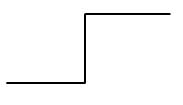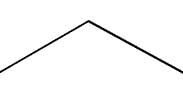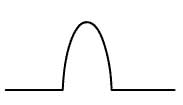Step edge Roof edge "Edge"

Roof edges rarely happen in practical cases so the focus will be on step edges.

Flat edge: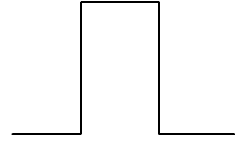Flat edge: combination of two step edges

Edge Detection I

The Human Visual System is very sensitive to details (ex: abrupt changes, edges). These details in the Fourier or frequency domain are located in the high frequencies.

One way to detect edges or variations within a region of an image is by using the gradient operator. For instance, the gradient, G, is a vector with two elements, Gx and Gy, where Gx is the gradient in the width direction and Gy is the gradient in the height direction. Since G is a vector, its magnitude Gm and direction Theta are given by: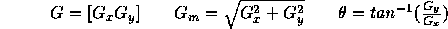There are several well known gradient filters. In this experiment we use the Sobel gradients, which are obtained by convolving the image with the following kernels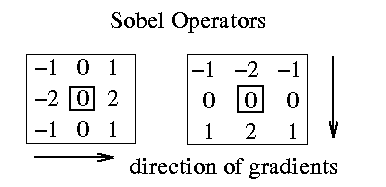Gx is the kernel on the right and Gy the one on the left. The kernel origin is located at the center and the arrows indicate the direction that each kernel measures. That is, the direction of Gx is from left to right (west-to-east), and Gy is from top to bottom (north-to-south).

a)Original image; b)Sobel Edge Detector (Gm)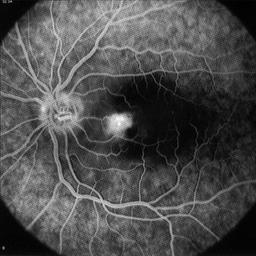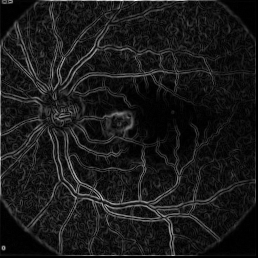a) b)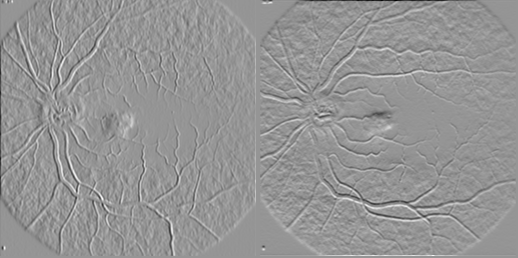In general, each kernel computes the gradient in a specific direction and later these partial results are combined to produce the final result. Each partial result computes an approximation to the true gradient by either using Euclidean distances or absolute differences. Absolute value computations are faster operations when compared to square and square root operations, thus, a faster way of computing the gradient magnitude is to sum the absolute values of the gradients in the X (width) and in the Y (height) directions.

Edge Enhancement

Edge enhancement is a method to accentuate the edges of an image. Edges are those pixels which lie on the boundary of two different values. The strength of the edges is determined by the difference between the two values adjacent to the edge.

Edge enhancement can be achieved by spatially convolving some region of the image with a mask. A mask is a set of weighting values. For example, consider the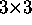mask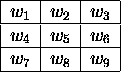where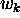is a weighting value for the mask. Also, consider an equally sized region within the image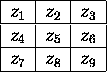where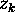is the value of a particular pixel. The response of this mask on the image is calculated by summing the products of the mask coefficients and the corresponding image pixel values,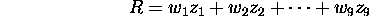These formulas can be expanded to operate on larger masks. In general, for an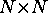mask, the response R is calculated as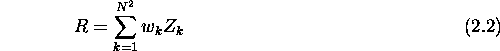In order to perform edge detection, masks such as these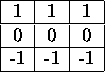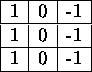can be used to perform horizontal and vertical edge enhancement respectively. Each mask is applied to the entire image and the resulting response is stored in an output array corresponding to the pixel located at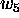and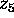.

Edge Detection

Image analysis deals with techniques for extracting information from images. The first step is generally to segment an image. Segmentation divides an image into its constituent parts or objects. For example in a military air-to-ground target acquisition application one may want to identify tanks on a road. The first step is to segment the road from the rest of the image and then to segment objects on the road for possible identification. Segmentation algorithms are usually based on one of two properties of gray-level values: discontinuity and similarity. For discontinuity, the approach is to partition an image based on abrupt changes in gray level. Objects of interest are isolated points, lines, and edges.

Definition of Edges

An edge is the boundary between two regions with relatively distinct gray-level properties. The idea underlying most edge-detection techniques is the computation of a relative derivative operator. The figure below illustrates this concept. The picture on the right shows an image of a light stripe on a dark background, the gray-level profile of a horizontal scan line, and the first and second derivatives of the profile. The first derivative is positive when the image changes from dark to light and zero where the image is constant. The second derivative is positive for the part of the transition associated with the dark side of the edge and negative for the transition associated with the light side of the edge. Thus the magnitude of the first derivative can be used to detect the presence of an edge in the image and the sign of the second derivative can be used to detect whether a pixel lies on the light or dark side of the edge.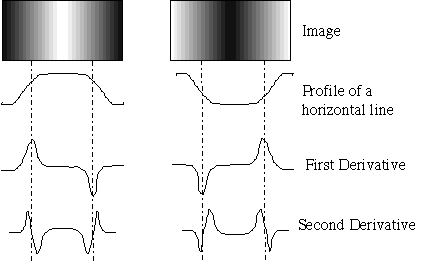We will use two dimensional spatial masks to find lines of different directions in an image. The mask is similar to the filters used in part 2 of this lab except that it operates in two dimensions. The mask is centered upon each pixel in the image and the following weighted calculation is performed (x is the original image pixels, y is the output):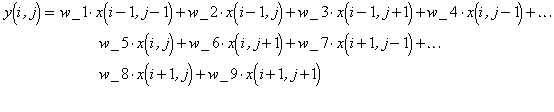w_1 w_2 w_3 w_4 w_5 w_6 w_7 w_8 w_9

The masks shown below respond more strongly to lines of different directions in an image. The first mask would respond very strongly to horizontal lines with a thickness of one pixel. The maximum response would occur when line passed through the middle row of the mask. The direction of a mask can be established by noting that the preferred direction is weighted with a larger coefficient that the other possible directions.

(a)

 -1 -1 -1 2 2 2 -1 -1 -1

(b)

 -1 -1 2 -1 2 -1 2 -1 -1

(c)

 -1 2 -1 -1 2 -1 -1 2 -1

(d)

 2 -1 -1 -1 2 -1 -1 -1 2

Exercises Directional Edge Detection

2. Filter the image using filters (a), (b),) above. Describe your results for each case. Which edges were highlighted in each case? The matlab commands for the first case are given below.
>wa=[-1,-2,-1;0,0,0;1,2,1];
>y_a=spfilt(f16,wa);
>y_a=round(y_a);
>imagesc(y_a);colormap('gray');
1. Combine the output of the images by adding together the absolute values of the edge. Save your results by plotting the four output images together.

The gradient of an image is the vector, del(f) shown below. The gradient vector points to the direction of maximum rate of change of f at (x,y). In edge detection the important quantity is the magnitude of this vector which is usually referred to as the "gradient." The gradient is the maximum rate of increase of f(x,y) per unit distance in the direction of the gradient vector. This vector is commonly approximated with absolute values as shown below.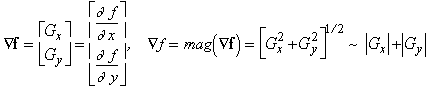Derivatives may be implemented in digital form in many different ways. The Sobel operators given below provide a differentiating and smoothing effect. Computation of the gradient at the location at the center of the masks using the equations given below gives the value of the gradient. To get the next value the mask is moved to the next pixel location and the procedure is repeated.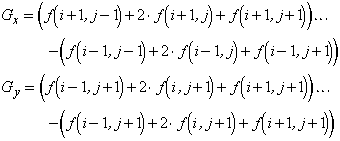Gx

 -1 -2 -1 0 0 0 1 2 1

Gy

 -1 0 1 -2 0 2 -1 0 1

1. Use the image lenna.256 as in the previous section, apply the mask G_x using the matlab files spfilt.m and spfilter.m . Save the result.
3. Calculate the Gradient image using the approximation given above (the sum of the absolute values of the gradients.
4. Plot the original image along with the gradient images on the same plot. Comment on the results for each mask, what types of edges gave a good response to reach filter, what happens when the results for Gx and Gy are combined in the gradient image? Compare the results with the edges from the previous section.
>G_x=[-1,-2,-1;0,0,0;1,2,1];
>G_y=[-1,0,1;-2,0,2;-1,0,1];
1. Load the image boatgn.256. Calculate the gradient of the image using the masks G_x and G_y. What happens to the edge detection results when the image is noisy? Would this technique be effective for noisy images?

The material in this lab is based on section 7.1 of the book Digital Image Processing, by R. C. Gonzalez and R. E. Woods, Addison-Wesley:Reading, MA, 1992.

An edge in an image can be describe by the derivative along x and y of the edge and the direction of the edge, as shown in the figure below.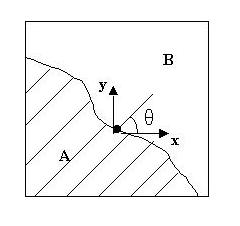The derivative and second derivative of such an edge are shown in the figure below.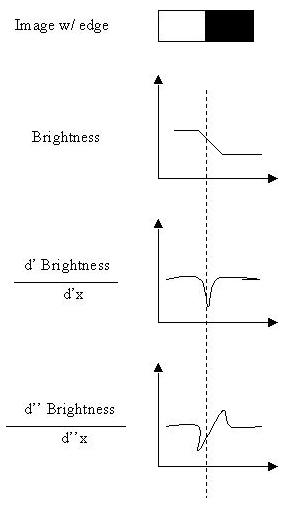To find the derivative of an edge in a discrete system the gradient of the edge will suffice. In this assignment we are using a weighted gradient average using the Sobel operator shown below.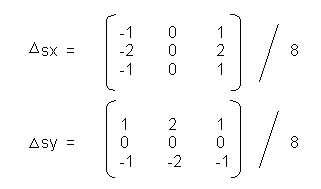The purpose of this assignment was to implement edge detection using this method and experiment with different thresholding methods using the gradient and direction of the edge image. The first step was to find dx and dy by summing the values of the 3x3 window multiplied by the sobel operator values. Then once the dx and dy was found for a particular pixel the gradient and the direction could be found for the pixel using these formulas,

direction = atan (dy/dx)

The image that is displayed as the edge detected image is the original gradient image. We were then to implement some different thresholding techniques in order to make the edges more distinguishable. An example is shown below with both the butterfly and lax pgm images.Original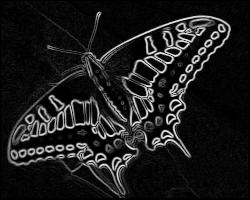Edge Image of Original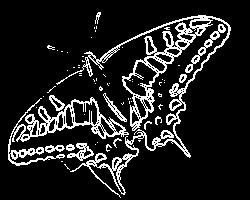Threshold of Edge Image - Gradient at 78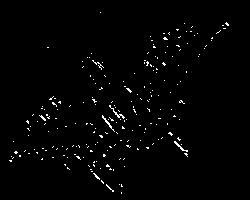Threhold of Edge Image - Gradient at 78, Direction at 45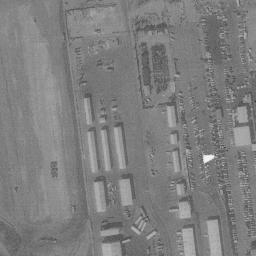Original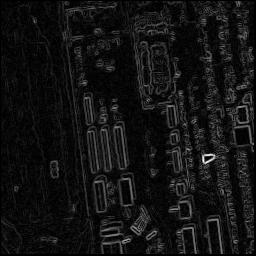Edge Image of Original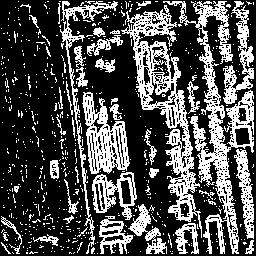Threshold of Edge Image - Gradient at 20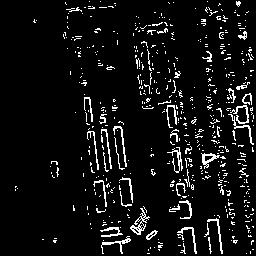Threshold of Edge Image - Gradient at 56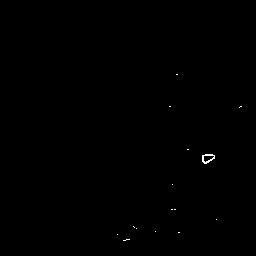Threshold of Edge Image - Gradient at 129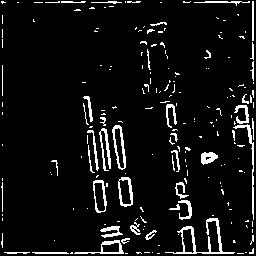Threshold of Edge Image after Original was Gaussian Smoothed - Gradient at 50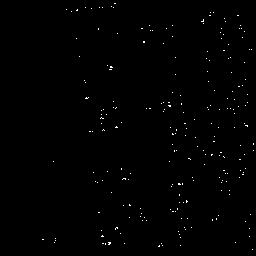Threshold of Edge Image - Gradient at 55, Direction at 0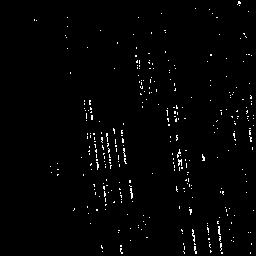Threshold of Edge Image - Gradient at 55, Direction at 90

These images show what drastic differences thresholding can make to the detection of the edge. If the threshold is too low the image is kind of noisy with edges. If the threshold is too high, not enough edge information can be seen. However, if the threshold is within the proper range it can show the edges rather well. For example, the butterfly image thresholded at 78 and the lax image thresholded at 56. When using the direction of the edge we can filter out all directions so that you can see just horizontal lines or vertical lines or any other direction that you deem useful to view. As seen in the above image that was gaussian smoothed before edge detection, the smoothing has an interesting result on the edge detection of the image. The edges of the buildings were more pronounced with the gaussian smoothing and seem a bit thicker. You would expect the same results if the image where passed through a low-pass filter and an edge detector, thicker and less noisy edge lines.